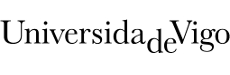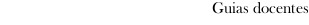Educational guide 2017_18Escola de Enxeñaría Industrial
 Degree in Industrial Technologies EngineeringSubjectsMechanics of materialsContents
 Topic Sub-topic 1. Introduction 1.1 Introduction 1.2 Review of statics fundamentals and applied concepts for further progress in solid mechanics and stress analysis 2. Axial load 2.0 Stress and strain. Linear elastic materials 2.1. Normal stress in an axially loaded prismatic bar. 2.2. Equilibrium of a deformable body. 2.3. Stress-Strain diagram of ductile materials. Hookes Law. 2.4. Elastic deformation of an axially loaded member. 2.5. Saint-Venant principle and superposition principle. 2.6. Statically governed problems. 2.7. Statically indeterminate problems. 2.8. Thermal stress and assembly misfits. 3. Bending 3.1 Beams: definition and types. Loads on beams. 3.2 Internal shear forces and bending moments. 3.3 External load, shear force and bending moment relationships. 3.4 Shear and moment diagrams 3.5 Pure bending and non-uniform bending. Hypothesis and limitations. 3.6 Normal stresses in unsymmetric bending. 3.7 Symmetric bending. The flexure formula (Naviers Law). 3.8 Section modulus of a beam. Ideal beam cross-section. 3.9 Deflection of beams and shafts. Rotation and displacement. Mohrs Theorems. 3.10 Statically indeterminate problems. 4. Buckling of columns 4.1. Critical load 4.2. Ideal column with pin supports 4.3. Columns having different types of supports. 5. Transverse Shear 5.1 Shear in straigh members 5.2. The shear formula 5.3. Design of joints. 6. Torsion 6.1. Torsional deformation of a circular shaft 6.2. The torsion formula 6.3. Angle of twist
 Universidade de Vigo            | Reitoría | Campus Universitario | C.P. 36.310 Vigo (Pontevedra) | Spain | Tlf: +34 986 812 000